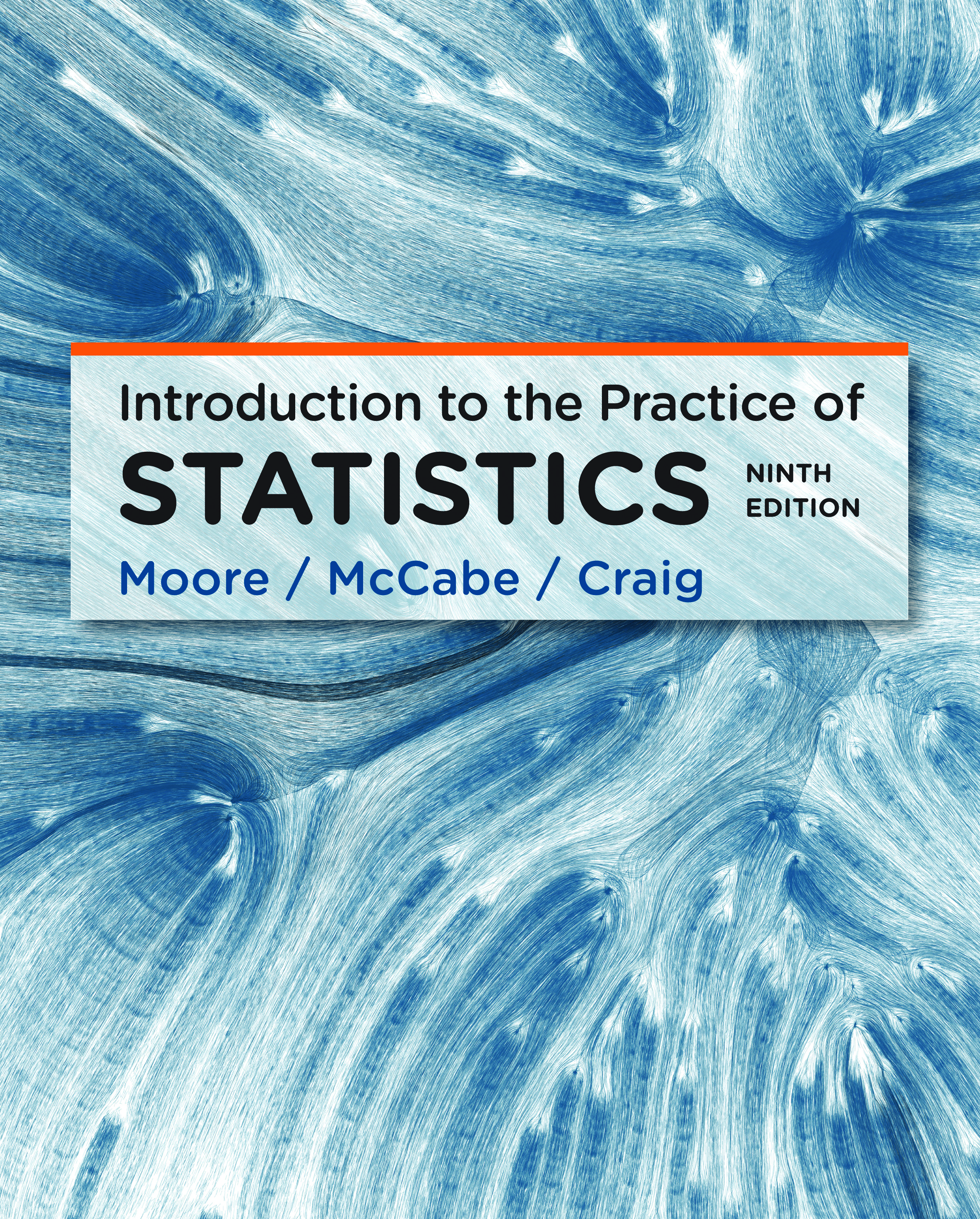# Moore/McCabe/Craig Introduction to the Practice of Statistics Ninth Edition

David Moore and George McCabe transformed educational statistics by writing a text that elevates systematics ways of thinking about data over mere techniques and formulas. As the title suggests, Introduction to the Practice of Statistics (IPS) introduces readers to statistics as it is used in practice. To this end, statistics is treated as the science of data. The authors present methods of basic statistics in a way that emphasizes working with data and mastering statistical reasoning, problem solving (rather than on methods), and drawing conclusions from data.## IPS 9e

• The focus is on the production and analysis of data as well as the traditional topics of probability and inference.

• Data production and data analysis are a major focus, while inference is treated as a tool that helps to draw conclusions from data in an appropriate way.

• The authors emphasize systematic ways of thinking about data.

• Students learn the technique of least-squares regression and how to interpret the regression slope. They also learn the conceptual ties between regression and correlation and the importance of looking for influential observations.

• Brief, optional "Beyond the Basics" sections give quick overviews of topics such as density estimation, scatterplot smoothers, data mining, nonlinear regression, and meta-analysis. Chapter 16 gives an elementary introduction to the bootstrap and other computer-intensive statistical methods.

• The authors focus on formulating questions, collecting and finding data, evaluating the quality of data, exploring the relationships among variables, performing statistical analyses, and drawing conclusions.

• The methods presented on real sets of data provide students with an excellent understanding of statistical concepts, homing in on the input and the output for a statistical analysis.

• The authors' inclusion of real data, rather than artificial data chosen to illustrate a calculation, serves to motivate students and help them see the usefulness of statistics in everyday life.

• Statistics is presented as something to do rather than something to talk about, and throughout the text students perform some relatively simple tasks that reinforce the material just presented.

• The authors capitalize on software to concentrate efforts on the big picture: what questions are we trying to address with a study and what can we conclude from our analysis?

• Students using IPS will be well prepared to undertake projects, oral presentations, article critiques, and written reports.